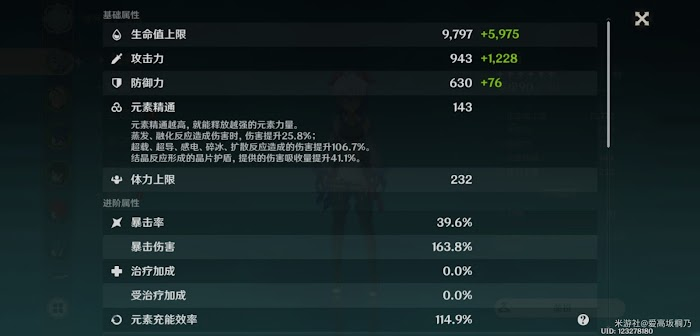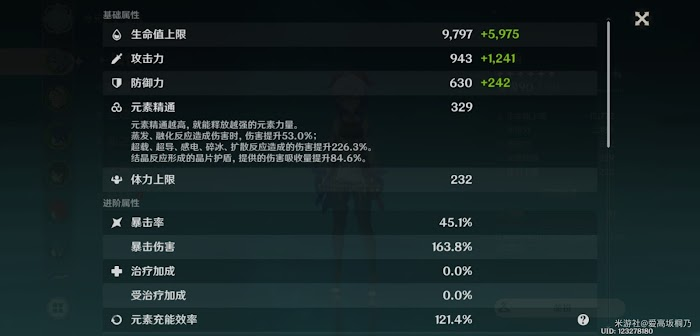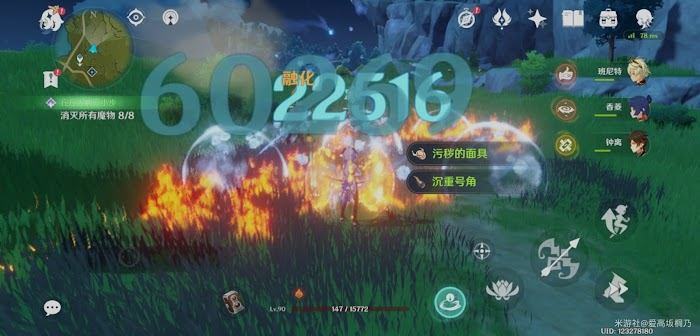# 原神 - 爆傷與暴擊期望資料計算30×4+60+20+60+40=300

①50%爆率，200%爆傷

②60%爆率，180%爆傷

③70%爆率，160%爆傷

④80%爆率，140%爆傷

①E（x）=0.5＋3×0.5=2

②E（x）=0.4+2.8×0.6=2.08

③E（x）=0.3+2.6×0.7=2.12

④E（x）=0.2+2.4×0.8=2.12